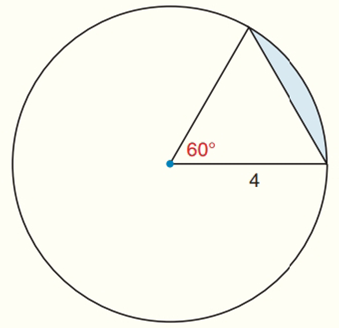Chapter 8.CR, Problem 29CR### Elementary Geometry for College St...

6th Edition
Daniel C. Alexander + 1 other
ISBN: 9781285195698

#### Solutions

Chapter
Section### Elementary Geometry for College St...

6th Edition
Daniel C. Alexander + 1 other
ISBN: 9781285195698
Textbook Problem
3 views

# Find the exact areas of the shaded regions in Exercises 27 to 31.To determine

To find:

The exact areas of the shaded regions.

Explanation

Calculation:

Sketch the following diagram:

The area of right angle triangle A=12bh

To find height:

The altitude, h, divides the equilateral into two 30-60-90-degree right triangles and divides the bottom side in half.

Long leg of the triangle = short leg ×3

To find short leg (a) of the triangle:

2a=42a2=42a=2

Long leg of the triangle (a3) = 23

Height (h) = 23 and base (b) = 4

To find the area right angle triangle:

### Still sussing out bartleby?

Check out a sample textbook solution.

See a sample solution

#### The Solution to Your Study Problems

Bartleby provides explanations to thousands of textbook problems written by our experts, many with advanced degrees!

Get Started

#### Evaluate: 123(52)

Elementary Technical Mathematics

#### Use Definition 8 to prove that limx1x=0. Definition 8

Single Variable Calculus: Early Transcendentals, Volume I

#### Prove Equation 4.

Single Variable Calculus: Early Transcendentals

#### True or False: converges.

Study Guide for Stewart's Multivariable Calculus, 8th

#### Evaluate: 12x21xdx. a) 322 b) 2+21 c) 4+2 d) 14

Study Guide for Stewart's Single Variable Calculus: Early Transcendentals, 8th